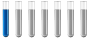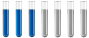## Molecular Formula

Chemistry and homework help forum.

Organic Chemistry, Analytical Chemistry, Biochemistry, Physical Chemistry, Computational Chemistry, Theoretical Chemistry, High School Chemistry, Colledge Chemistry and University Chemistry Forum.

Share your chemistry ideas, discuss chemical problems, ask for help with scientific chemistry questions, inspire others by your chemistry vision!

Please feel free to start a scientific chemistry discussion here!

Discuss chemistry homework problems with experts!

Ask for help with chemical questions and help others with your chemistry knowledge!

Moderators: expert, ChenBeier, Xen

yabasic
NewbiePosts: 1
Joined: Wed Sep 01, 2021 3:02 pm

### Molecular Formula

Lactic acid is a ternary oxyacid that is 40.00% carbon and 6.67% hydrogen. If 0.377 moles of lactic acid have a mass of 33.93 g, find the molecular formula of lactic acid.

So I have the answer (C3H6O3) but I don't understand how to solve it. Can you help me?
ChenBeier
Distinguished MemberPosts: 903
Joined: Wed Sep 27, 2017 7:25 am
Location: Berlin, Germany

### Re: Molecular Formula

Use n= m/M, solve to M to get molecular weight.
This calculate with the given percentage.
Divide each result through the molecular mass of the elements.
Warner Mortensen
Full MemberPosts: 15
Joined: Mon Jul 26, 2021 4:13 am

### Re: Molecular Formula

Molecular formula provides us with the number of atoms present in each element of a molecular substance or molecular compound. Molecular formula presents the actual ratio between the atoms of a compound.
Formula:
Molecular formula = (empirical formula)n
N = M/empirical formula mass

In this question to know the molecular formula of Lactic Acid, it is important to know its empirical formula as well because molecular formula is linked with empirical formula.
Empirical Formula is found like this,
Carbon Hydrogen Oxygen
40/12 6.67/1 53.3/16
3.3 6.67 3.33 3.3 is the smallest value that will be used to divide all the values obtained
3.3/3.3 6.67/3.3 3.33/3.3
1 2.02 1.009
C H2 O1 is the empirical formula of Lactic Acid

E.F mass = 12x1 + 1x2 + 16x1
Mole = mass/ Mr
0.377 = 33.93/Mr
Mr =90
N= 90/30 n = 3
Now divide the empirical formula with n value
(C H2 O1) x3
C3 H6 03 is the answer.

Hence, C3 H6 03 is the molecular formula of Lactic acid.